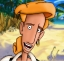# SannyBuilder.com Forums

You are not logged in.

## #1 31-03-2008 20:12

AschrattFrom: Chemnitz
Registered: 07-11-2007
Posts: 8
Website

### CLEO Sources

Hey!

I thought a thread where you can post some generic sources would be cool and useful.
So I start with a source to read a number out of a textfile.
Generaly it converts a string to an integer... You can modify this to get a StrToI function.

Notes: Create a file and write a number in ".." (Example: "123") it. The Source finds out the start (") and reads the number. if it founds " it returs the read numbers as an integer. If it founds an illegal character it should simply continue calculation.

```// --== SCM STRING TO INT CONVERTER ==--
// ---===  (C)2008 - by Aschratt  ===---

// This function reads a value in ".." out of a file and returns the number as an integer

{\$CLEO}

0050: gosub @CONVERT

//Additional Source. 10@ contains the value now.

:CONVERT
0006: 0@ = 0        //File handle
0006: 10@ = 0       //Read buffer
0006: 1@ = 0        //result

:CONVERT_START
0A9A: 0@ = openfile ".\CLEO\blah.ini" mode 0x6272   // Binary Read (TextReading only used for complete strings)

:CONVERT_LOOP_01                                    //Searching " for starting
0001: wait 0 ms
0A9D: readfile 0@ size 1 to 10@                     // store 1 byte to the buffer
00D6: if 0
0039:   10@ == 34                                   // '"'
004D: jump_if_false @CONVERT_LOOP_01

:CONVERT_LOOP_02
0001: wait 0 ms
0A9D: readfile 0@ size 1 to 10@                     // store 1 byte to the buffer
00D6: if 0
8039:   not 10@ == 48                               // '0'
004D: jump_if_false @CONVERT_CASE_0
00D6: if 0
8039:   not 10@ == 49                               // '1'
004D: jump_if_false @CONVERT_CASE_1
00D6: if 0
8039:   not 10@ == 50                               // '2'
004D: jump_if_false @CONVERT_CASE_2
00D6: if 0
8039:   not 10@ == 51                               // '3'
004D: jump_if_false @CONVERT_CASE_3
00D6: if 0
8039:   not 10@ == 52                               // '4'
004D: jump_if_false @CONVERT_CASE_4
00D6: if 0
8039:   not 10@ == 53                               // '5'
004D: jump_if_false @CONVERT_CASE_5
00D6: if 0
8039:   not 10@ == 54                               // '6'
004D: jump_if_false @CONVERT_CASE_6
00D6: if 0
8039:   not 10@ == 55                               // '7'
004D: jump_if_false @CONVERT_CASE_7
00D6: if 0
8039:   not 10@ == 56                               // '8'
004D: jump_if_false @CONVERT_CASE_8
00D6: if 0
8039:   not 10@ == 57                               // '9'
004D: jump_if_false @CONVERT_CASE_9
00D6: if 0
8039:   not 10@ == 34                               // '"'
004D: jump_if_false @CONVERT_END
0002: jump @CONVERT_LOOP_02                         // illegal character -> GetNext

:CONVERT_END
0A9B: closefile 0@
0051: return

:CONVERT_CASE_0
0012: 1@ *= 10                                      // Add one position to the end number
000A: 1@ += 0                                       // The number which was found
0002: jump @CONVERT_LOOP_02

:CONVERT_CASE_1
0012: 1@ *= 10                                      // Add one position to the end number
000A: 1@ += 1                                       // The number which was found
0002: jump @CONVERT_LOOP_02

:CONVERT_CASE_2
0012: 1@ *= 10                                      // Add one position to the end number
000A: 1@ += 2                                       // The number which was found
0002: jump @CONVERT_LOOP_02

:CONVERT_CASE_3
0012: 1@ *= 10                                      // Add one position to the end number
000A: 1@ += 3                                       // The number which was found
0002: jump @CONVERT_LOOP_02

:CONVERT_CASE_4
0012: 1@ *= 10                                      // Add one position to the end number
000A: 1@ += 4                                       // The number which was found
0002: jump @CONVERT_LOOP_02

:CONVERT_CASE_5
0012: 1@ *= 10                                      // Add one position to the end number
000A: 1@ += 5                                       // The number which was found
0002: jump @CONVERT_LOOP_02

:CONVERT_CASE_6
0012: 1@ *= 10                                      // Add one position to the end number
000A: 1@ += 6                                       // The number which was found
0002: jump @CONVERT_LOOP_02

:CONVERT_CASE_7
0012: 1@ *= 10                                      // Add one position to the end number
000A: 1@ += 7                                       // The number which was found
0002: jump @CONVERT_LOOP_02

:CONVERT_CASE_8
0012: 1@ *= 10                                      // Add one position to the end number
000A: 1@ += 8                                       // The number which was found
0002: jump @CONVERT_LOOP_02

:CONVERT_CASE_9
0012: 1@ *= 10                                      // Add one position to the end number
000A: 1@ += 9                                       // The number which was found
0002: jump @CONVERT_LOOP_02```

Offline

## #2 01-04-2008 14:43

SeemannRegistered: 07-08-2006
Posts: 2,050

### Re: CLEO Sources

Good, but the code need to be optimized.

How 'bout making a routine that will read a word from the file (until it meets a space sign #32), and store this word to a string variable. Say, I have a file with

`Hello, world!`

inside. Then I run this routine and it will store Hello, to some variable. The routine could have an additional parameter to specify which word I need: first, second, third, etc. In my example, Hello, is the first word  and world! is the second. Also it could optionally skip everything except letters and numbers.

It will be useful to make a real routine StrToInt, that converts a string variable value to a number.
Then, to get a number from the file, I will run first routine with parameter 1, 2, etc and then the StrToInt.

Offline